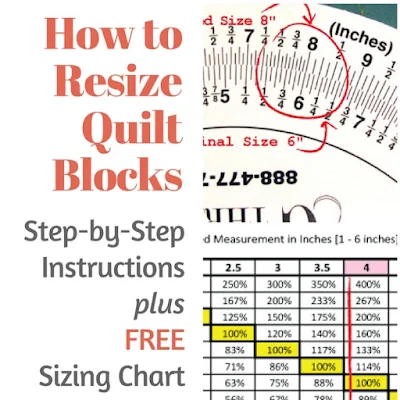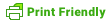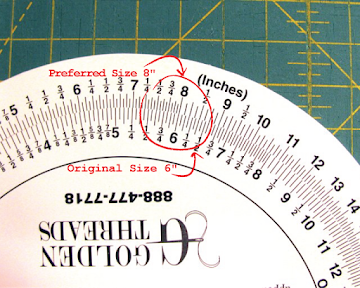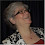### How to Resize Quilt Blocks and PatternsQuilt patterns can come in many sizes. But what if the size of the pattern isn't the size you want? Maybe you want a throw instead of a mini quilt or a baby quilt instead of a king-size. To resize your pattern or block, you need to know the percentage to reduce or enlarge the pattern templates. Read below to learn three easy ways to get the percentage you need to resize templates.

THREE METHODS FOR RESIZING YOUR QUILTS AND BLOCKS

1. DO THE MATH
Below is the very simple formula to get the percentage for reducing or enlarging.

ENLARGING:

Measure the shortest length of the original block size? e.g. 6 inches
What size do you want the new block to be? e.g. 8 inches
Divide the new size by the original size, e.g. 8 ÷ 6 = 1.33.
Move the decimal point two steps to the right to get your percentage = 133%.
Enter 133% into the copy machine to enlarge your 6-inch block to an 8-inch block.

REDUCING:

Do the opposite of above.
Divide the small size (e.g. 6") by the large size (e.g. 8") to get your reduction
percentage, e.g. 6 ÷ 8 = 0.75 = 75% reduction.

2. PROPORTIONAL SCALE
Proportional Scales may look a bit intimidating at first but they're easy to use.
You can buy a Proportional Scale for quilters HERE.How To Use a Proportional Scale
1. On the bottom wheel, find the original size of your block (e.g. 6"), [Fig 1].
2. Line up this number with the new size (e.g. 8") on the top wheel, [Fig 1].
3. In the window, you'll see the percentage of the reduction or enlargement you need to resize your block, [Fig 2].
Note: The math formula and proportional scale results may be off a tiny bit. This is okay.Figure 1Figure 2

3. PROPORTIONAL MEASUREMENT CHART
2. Find the original measurement on the left side of the chart.
3. Go along that row until you get to the measurement you want on the top row.
4. The number in the intersecting box is the percentage you'll need to reduce or enlarge your block1.Thank you soooo much! This is what I have been seeking. You ROCK!

1.You're very welcome Nycki. No one ever told that I rocked before. LOL.

2.This is perfect! I NEVER use a pattern, but sometimes a combination of two or more ideas. This really helps in designing and changing that baby pattern into a queen! Thank you

3.Good grief! Math!

1.I think the chart is the easiest of the three.

4.Thank you so much for this!

5.Thank you so much for this!

6.As a fairly new quilter this is a game changer! It evens the playing field so to speak. Thank you!